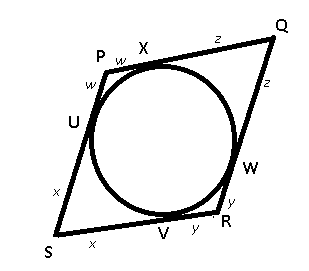### XAT 2011 Question 93

Instructions

are followed by two statements labelled as I and II. Decide if these statements are sufficient to conclusively answer the question. Choose the appropriate answer from the options given below:

A. Statement I alone is sufficient to answer the question.
B. Statement II alone is sufficient to answer the question.
C. Statement I and Statement II together are sufficient, but neither of the two alone is sufficient to answer the question.
D. Either Statement I or Statement II alone is sufficient to answer the question.
E. Neither Statement I nor Statement II is necessary to answer the question.

Question 93

# Let PQRS be a quadrilateral. Two circles O1 and O2 are inscribed in triangles PQR and PSR respectively. Circle O1 touches PR at M and circle O2 touches PR at N. Find the length of MN.I. A circle is inscribed in the quadrilateral PQRS.II. The radii of the circles O1 and O2 are 5 and 6 units respectively.

Solution

Using the property that, tangents from same point to a circle are equal in lengths.

In above quadrilateral, PA = PM + MN, => $$d = a + MN$$ ----------Eqn(I)

RC = RN + NM

=> $$PS = d + e$$

$$SR = e + c$$

$$QR = b + c + MN$$

$$PQ = a + b$$

From statement I :We can conclude that, $$w + x + y + z = w + x + y + z$$

=> $$(w + z) + (x + y) = (w + x) + (y + z)$$

=> $$PQ + SR = PS + QR$$

Substituting values from above equation, we get :

$$\therefore a + b + e + c = d + e + b + c + MN$$

Using eqn(I),

=> $$a = a + MN + MN$$

=> $$MN = 0$$

Thus, statement I alone is sufficient.

Statement II alone is not sufficient, for we can have more than one value of MN possible.